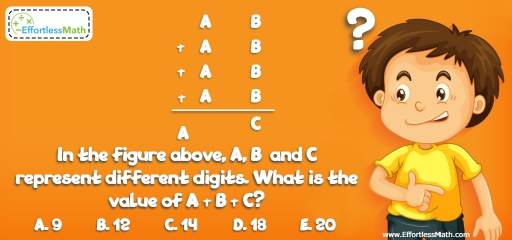# Intelligent Math Puzzle – Challenge 87

Another mind-blowing math puzzle to measure your critical thinking skills. If you couldn't solve it, take it easy! It is a challenging problem for even the smartest people!## Challenge:

In the figure above, A, B and C represent different digits. What is the value of A + B + C?

A- 9

B- 12

C- 14

D- 18

E- 20

### The Absolute Best Book to challenge your Smart Student!

A, B and C represent different digits. Plug in different digits for B and find the value of A. Remember that the sum of four 2-digit numbers is another 2-digit number. Therefore, the value of A cannot be more than 2. Why?
A is 1 or 2. The value of 1 for A is not possible. Because the sum of four same numbers (B) cannot be 1, 11 or 21, and 31.
A is 2. So, B can be 3 or 8.
B cannot be 8. Because 4 times 28 is 112, which is not a 2-digit number.
B is 3. Then, C is 9.
A + B + C = 2 + 3 + 9 = 14

### What people say about "Intelligent Math Puzzle – Challenge 87 - Effortless Math: We Help Students Learn to LOVE Mathematics"?

No one replied yet.

X
30% OFF

Limited time only!

Save Over 30%

SAVE $5 It was$16.99 now it is \$11.99